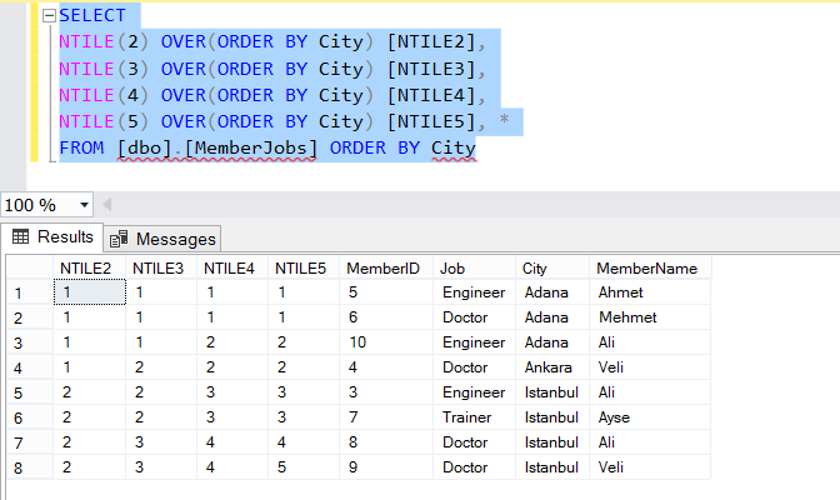Thursday , June 8 2023

# Ranking Functions(ROW_NUMBER, RANK, DENSE_RANK, NTILE) in SQL Server(TSQL)

These are the special sorting functions that we can use to sort the data that we select from the tables at run time.

• ROW_NUMBER
• RANK
• DENSE_RANK
• NTILE

First, create a table as follows and add a few records into it with the following script.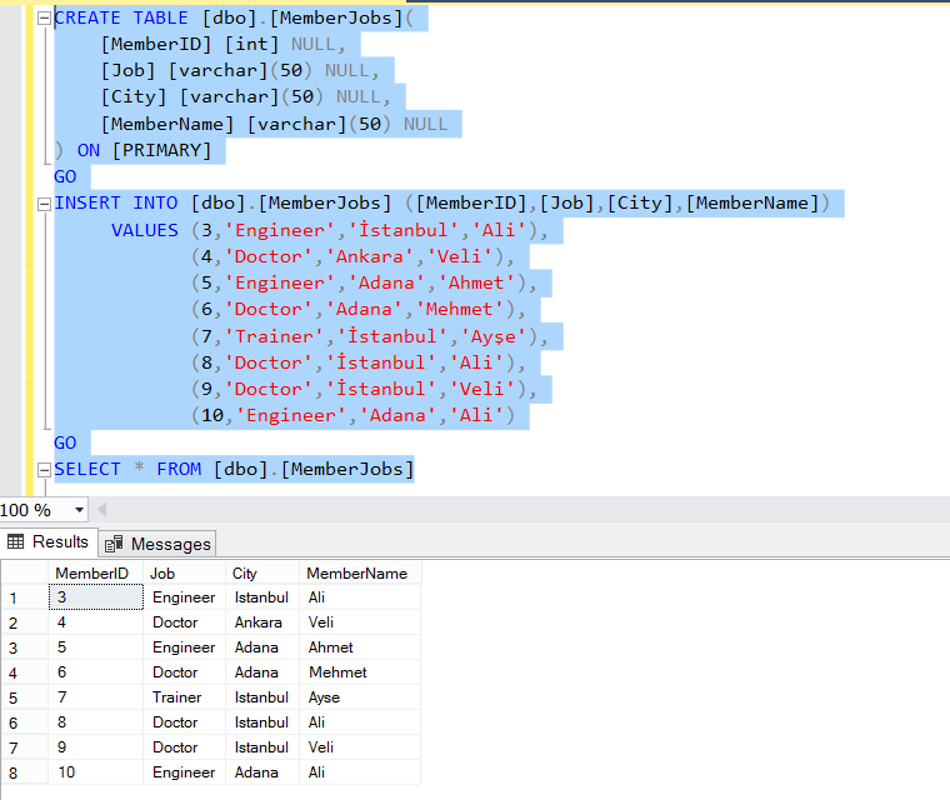### ROW_NUMBER

This function sequentialy numbers each row starting from 1 according to the sort expression.

Number the output by sorting the City and MemberId columns.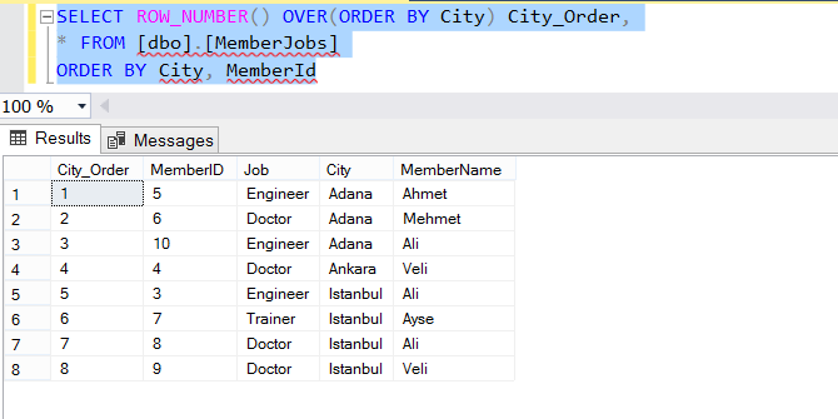We can sort the different data in a column separately.  Let’s sort the cities depending on the Job column according to the values ​​in the example.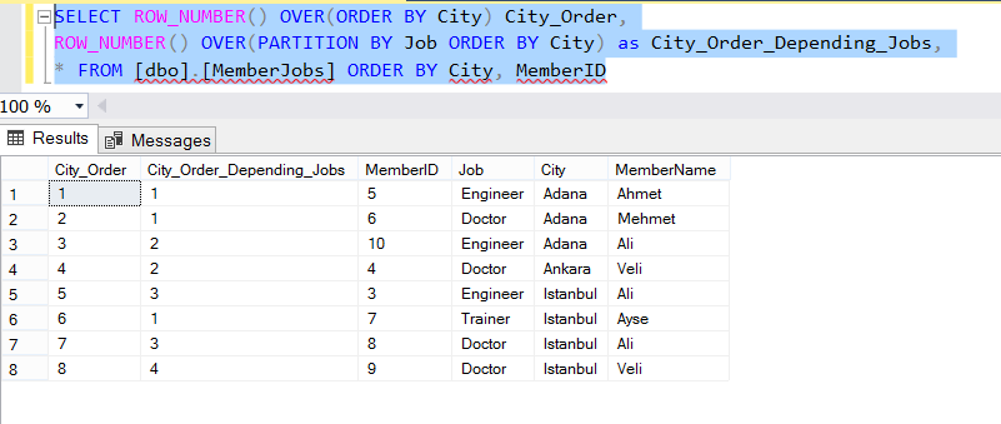### RANK and DENSE_RANK Functions:

The RANK () and DENSE_RANK () functions group data according to a column and sort the groups by assigning a number to each group.

The difference between RANK and DENSE_RANK functions:

In the RANK () function; While the value of the first group is 1, and the value of the second group is not always 2. If there are 3 values ​​in the first group, the value of the second group is 4. In the DENSE_RANK () function, the group numbers are consecutive. No matter how many records in the first group, the value of the second group is always 2. In the following example, this topic is very clear.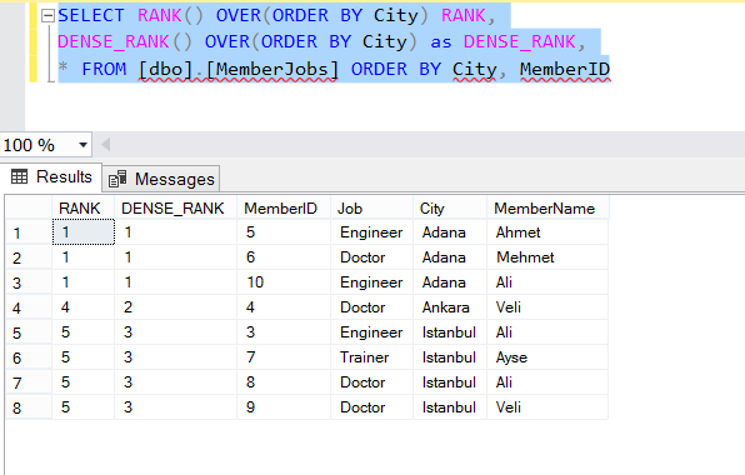### NTILE Function:

NTILE () will sort the dataset according to the columns specified by OVER and ORDER BY. And groups the records by the number specified in the parameter, and  gives each group a sequential number.

The integer_expression parameter is a positive integer indicating the number of groups. Suppose that 30 records are returned as a result of the query and the parameter value specified in the NTILE () function is 5. In this case, 5 groups of 6 rows are created. And each group is given sequential numbers, starting from 1. That is, the number of the first 6 records is set to 1, the number of the next 6 records is 2, and the number of the last 6 records is set to 5.

There are 8 records in our example, let’s try NTILE with different values.